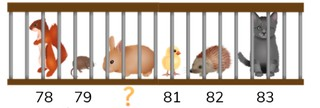Counting to 100 crossing tens

# Counting to 100 crossing tens

Students learn to count forward and backward to 100 crossing tens.

No account needed.8,000 schools use Gynzy92,000 teachers use Gynzy1,600,000 students use Gynzy

## General

Students are able to count forward and backward to 100 crossing tens. They know which numbers come before and after a given tens number.

-K.CC.A.1

## Relevance

Discuss with students that it is important to be able to count crossing tens, so you are able to easily count and can work on learning to calculate.

## Introduction

The interactive whiteboard has a selection of numbers. Point at a number and ask students to say the number out loud.

## Development

Start by reminding students that tens are a group of 10 that end with a 0 in the ones place. Explain to students that when you cross tens counting forward, that the number in the tens place goes up by one. So you count from 28 to 29 to 30 to 31. If you cross tens counting backward, the number in the tens place goes down by one. So you count from 31 to 30 to 29 to 28. Next show the number sequence from 8 to 12 with the digits in the tens place in blue. Count out loud, pointing at the numbers, and have students count along. Then show them the other sequences. Count these together out loud counting forward and backward. Next practice counting and crossing tens. Show a sequence of numbers and ask students to count forward, so they can determine which numbers to fill into the blanks. Have students write the numbers on a sheet of paper/mini-whiteboard and hold them up to check their work. Erase the grey squares to check their work. Have them practice this again, counting and writing the missing numbers.

Check that students can count forward and backward to 100 crossing tens by asking the following questions:
- What are tens?
- Which number comes after 79?
- Which number comes before 60?

## Guided practice

Students are given number sequences in which they must fill in the missing number(s) counting forward or backward, while crossing tens.

## Closing

Remind students that it is important to be able to count forward and backward to 100 crossing tens, so you can determine a number and learn to calculate. Check that they can explain what tens are. Ask students to write the missing numbers in the number sequence on a sheet of paper/mini-whiteboard and have them hold up their answers to check them. Then ask students to drag the missing numbers to the number sequences on the interactive whiteboard.

## Teaching tips

Students who have difficulty crossing tens are likely not yet comfortable with the tens numbers, nor with the number sequence. Ask them to first say the tens numbers, and then work your way slowly from one tens number to the next tens number counting one by one. Start by counting forward and then count backward.

## Instruction materials

Optional: mini-whiteboards

### The online teaching platform for interactive whiteboards and displays in schools

• Save time building lessons

• Manage the classroom more efficiently

• Increase student engagement